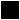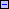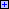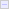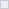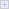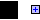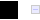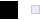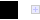CS216

Chris Pollett

Feb 16, 2010

Outline

• Bessel-Overhauser Splines
• Quiz
• TCB Splines
• B-Splines

Introduction

• Last day, we talked about using rational Bezier curves to represent conic sections.
• We then discussed rotating a Bezier curve to sweep out a surface of revolution and then how to get the corresponding Bezier patch.
• Finally, we talked about how to get a piece-wise degree three Bezier curve that interpolates (goes through) a set of points.
• Our first example, of how to do this was Catmull-Rom Splines. We next look at some other techniques for interpolation...

Bessel-Overhauser Splines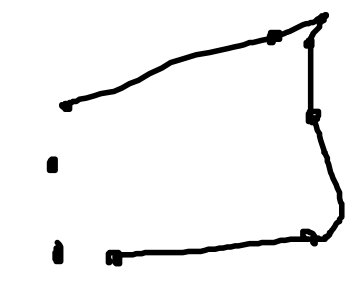• Recall the \vec L_i of last day for Catmull-Rom splines where defined in terms of the i-1st and i+1st control points. Because of this we can only define \vec p_i^+ and \vec p_i^- when 0 < i < m, so for Catmull-Rom Splines the first and last points are there to make the equations of the intermediate piecewise Bezier curves work out, but will not be interpolated.
• With Catmull-Rom splines, when knot points are not evenly spaced, you can get bad "overshoot" effects as seen above when two close control are next to widely separated control points.
• To solve this problem, one can use a chord-length parametrization. That is, we choose the knots so that u_(i+1) - u_i = ||\vec p_(i+1) - \vec p_i||.
• This will roughly make the arclength of the curve between \vec p_i and \vec p_(i+1) proportional to u_(i+1) - u_i.

More Bessel-Overhauser Splines

• To effect this parametrization, we need to modify our definitions of \vec p_i^- and \vec p_i^+ using the Bessel tangent method (aka the Overhauser method).
• Suppose we have a set of knots u_i. We can define the average velocity of the curve between \vec p_i and \vec p_(i+1) as
\vec v_(i+1/2) = \frac{\vec p_(i+1) - \vec p_(i)}{u_(i+1) - u_(i)}.
• If the chord length approximation was good, then these will be unit vectors, and we can approximate the velocity at \vec p_i as weighted average:
\vec v_i = \frac{(u_(i+1) - u_(i))\vec v_(i+1/2) + (u_(i) - u_(i-1))\vec v_(i-1/2)}{u_(i+1) + u_(i-1)}
• Finally, we can define the Bessel-Overhauser Splines, in the same way as Catmull-Rom splines but using \vec p_i^+ and \vec p_i^- defined by:
\vec p_i^(-) = vec p_i - \frac{1}{3}(u_i - u_(i-1))\vec v_i
\vec p_i^(+) = vec p_i + \frac{1}{3}(u_(i+1) - u_(i))\vec v_i

Quiz

Which of the following statements is true?

1. The glMap1* class of OpenGL functions can be used to set up a Bezier curve for drawing.
2. Rational Bezier curves do not make use of homogeneous coordinates.
3. All Catmull-Rom splines are parametrized over the unit interval.

Tension-Continuity-Bias Splines

• Sometimes a curve designer would like to have their curves be "tighter" to some control points and "looser" for others.
• TCB splines use three parameters: tension, continuity, and bias to allow a designer to have this kind of flexibility.
• Tension controls the tightness of the curve, continuity controls the discontinuity of the first derivatives, and bias controls the degree to which the curve undershoots or overshoots an interpolation point.
• The basic set-up begins again with Catmull-Rom Splines where we modify the definition of \vec p_i^+ and \vec p_i^-.
• We define:
\vec p_i^+ = \vec p_i +\frac{1}{3}D\vec q_i^+  and \vec p_i^(-) = \vec p_i -\frac{1}{3}D\vec q_i^-.
• D\vec q_i^\pm are supposed to represent the left and right derivatives of \vec q(u) at u_i. i.e., defined in terms of the limit as u approaches u_i from above for + and from below for -.

More TCB Splines

• For a basic Catmull-Rom spline D\vec q_i^\pm is equal to
D\vec q_i^(-) = D\vec q_i^(+) = \frac{1}{2}\vec v_(i-1/2) + \frac{1}{2}\vec v_(i+1/2)
where \vec v_(i-1/2) = p_i - p_(i-1).
• To add the tension effect we define:
D\vec q_i^(-) = D\vec q_i^(+) = (1-t)(\frac{1}{2}\vec v_(i-1/2) + \frac{1}{2}\vec v_(i+1/2))
• The continuity parameter is supposed to measure how close to C^1-continuous the curve is at \vec p_i.
• This can be modeled by defining:
D\vec q_i^(-) = \frac{1-c}{2}\vec v_(i-1/2) + \frac{1 + c}{2}\vec v_(i+1/2)
D\vec q_i^(+) = \frac{1+c}{2}\vec v_(i-1/2) + \frac{1 - c}{2}\vec v_(i+1/2)
Here c is usually set between -1 and 0.
• Finally, to model overshoot (what bias does) we can weight the two average velocities \vec v_(i-1/2) and \vec v_(i+1/2) differently by setting:
D\vec q_i^(-) = D\vec q_i^(+) = \frac{1+b}{2}\vec v_(i-1/2) + \frac{1-b}{2}\vec v_(i+1/2)
Here b is usually set between -1/2 and 1/2 where negative values yield undershoots, and positive ones yield overshoots.

TCB Splines All-in-One and Interpolation Conclusion

• Combining our three different models yields the following equations for D\vec q_i^\pm:
D\vec q_i^(-) = \frac{(1-t)(1-c)(1+b)}{2}\vec v_(i-1/2) + \frac{(1-t)(1+c)(1-b)}{2}\vec v_(i+1/2)
D\vec q_i^(+) = \frac{(1-t)(1+c)(1+b)}{2}\vec v_(i-1/2) + \frac{(1-t)(1-c)(1-b)}{2}\vec v_(i+1/2)
• The book shows how to generalize the interpolation problem from Bezier curves to Bezier patches.
• In the surface setting there are analogs to Catmull-Rom and Bessel-Overhauser curves.
• In the surface setting, one has to make use of the second derivatives for patches we defined earlier.
• We are going to skip this sections; however, and move on to B-splines.

B-splines

• We are now going to consider another way to define curves using control points: B-splines.
• B-splines were first defined in the 1940s, but gained popularity in the 1970's after good methods for defining their blending functions were discovered.
• B-spline curves have several advantages over Bezier curves in terms of modeling complicated curves with a single equations, allowing for sharp bends in a curve, and sensitivity to added control points.
• There are methods to translate back and forth between B-spline and piecewise Bezier curves.
• We will first look at the uniform B-splines and then consider nonuniform ones.

Uniform B-splines of Degree Three

• A uniform B-spline of degree three is defined by a sequence of control points \vec p_0, \vec p_1, \ldots, \vec p_n together with a set of blending functions N_0(u), N_1(u), \ldots, N_n(u) which parametrically define a curve via the equation:
\vec q(u) = \sum_(i=0)^n N_i(u)\cdot \vec p_i where 3 \leq u \leq n+1.
• We will define the blending functions in a moment, but one important property that have is that N_i(u) equals 0 if u \leq i or i+4 \leq u.
• This means if u \in [j, j+1], 3 \leq j \leq n, then the defining equation for \vec q(u) reduces to:
\vec q(u) = \sum_(i=j-3)^j N_i(u)\cdot \vec p_i.
• So a B-spline has local control, if a single control point \vec p_i is moved, then only the portion of the curve with i < u < i+4 is changed.
• Next day, we will discuss our requirements on what the N_i(u)'s can be.